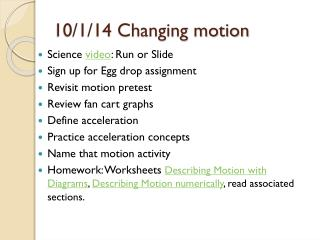DownloadDownload Presentation10/1/14 Changing motion

# 10/1/14 Changing motion

Download Presentation## 10/1/14 Changing motion

- - - - - - - - - - - - - - - - - - - - - - - - - - - E N D - - - - - - - - - - - - - - - - - - - - - - - - - - -
##### Presentation Transcript

1. 10/1/14 Changing motion • Science video: Run or Slide • Sign up for Egg drop assignment • Revisit motion pretest • Review fan cart graphs • Define acceleration • Practice acceleration concepts • Name that motion activity • Homework: Worksheets Describing Motion with Diagrams, Describing Motion numerically, read associated sections.

2. Learning objectives • Apply the definition of acceleration to solve for an unknown • Interpret the slope of a velocity vs time graph • Give a verbal description of the motion of a moving object

3. Revisit Motion pretest • Does today’s activity help us answer any of the Motion pretest items? • How do we know if there is acceleration? • When the velocity does not change. • Item 6 on the pretest asks about this. • Let’s look at yesterday’s graphs

4. Sample Velocity vs. time graph • The line equation is y = mx + b • What are y, x, m, b? • Here y is velocity, x is time, m describes the rate of speeding up, and b is initial velocity

5. Sample Position vs. time graph • What does each parameter represent here? • Look at similarities with the previous equation. • Here y is position, x is time, 8.1279 is 1/2a, 22.288 is initial velocity and 0.8107 is initial position.

6. Acceleration • The slope of a position vs. time graph is the velocity. • If the velocity is changing, the slope at each point represents the velocity at that point. • The slope of a velocity vs. time graph is the acceleration. • Definition of acceleration: Acceleration = change in velocity/change in time a=∆v/ ∆t (= rise of v vs t graph/run of v vs t graph) • The more the velocity changes for a set time interval, the greater the slope and the greater the acceleration. • Acceleration can be positive or negative • Review acceleration homework

7. Name That Motion activity • Go to http://www.physicsclassroom.com/shwave/namethat.cfm or http://goo.gl/yhw95 • Work in your table groups. Just enter one name. • View each motion. Copy down the pattern of dots for each motion. • Type the number of the description on the worksheet that matches the motion. • Staple the worksheet to your notebook.

8. Motion graphs for the activity